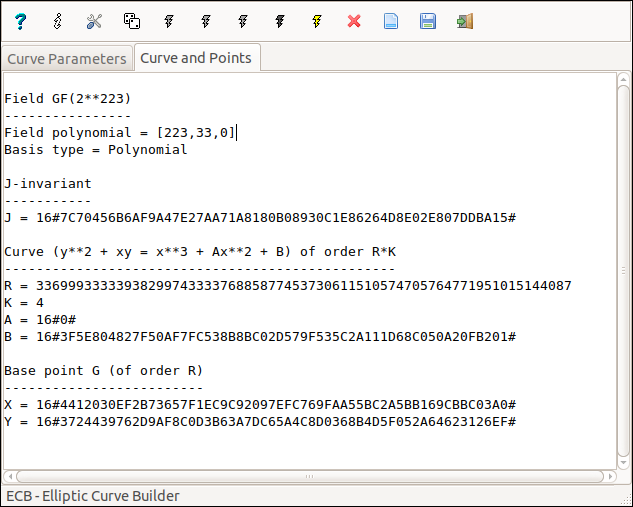Overview

ECB - Elliptic Curve Builder - is a generator of ordinary elliptic curves. The curves over the Galois fields GF(P), GF(2N) and GF(3N) are built using the so-called complex multiplication method. Even if, for any reason, one does not trust the curves produced with ECB, they remain useful in order to test and/or to tune ECC applications.Properties of a curve created with ECB

• over GF(P)
• equation y2 = x3 + Ax + B;
• the order is U = R*K with R prime and K < R;
• the binary size of the prime modulus P may be any in 30..1536.

• over GF(2N)
• equation y2 + xy = x3 + Ax2 + B;
• the order is U = R*K with R prime and K < R;
• the field degree N may be any in 30..1024;
• the basis of the field GF(2N) may be polynomial or normal.

• over GF(3N)
• equation y2 = x3 + Ax2 + B;
• the order is U = R*K with R prime and K < R;
• the field degree N may be any in 20..768;
• the basis of the field GF(3N) may be polynomial or normal.

Examples of use

Here are three examples of use with the three Galois fields:

Changes
v3.0.0  (January 13, 2020)
• Modified the Setup dialog box.
• Added the Random Seed dialog box.
• Miscellaneous internal improvements.
• Updated the help file.
Previous changes